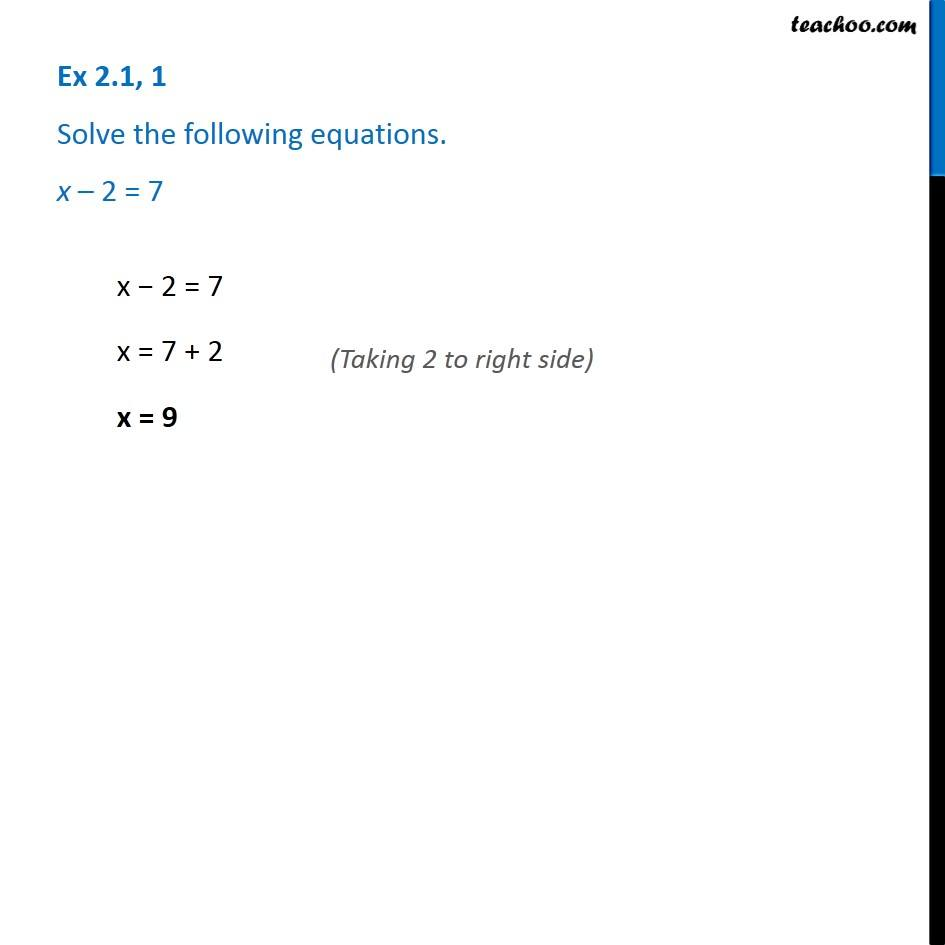Solving easy equations

Chapter 2 Class 8 Linear Equations in One Variable
Serial order wiseLearn in your speed, with individual attention - Teachoo Maths 1-on-1 Class

### Transcript

Question 1 - Chapter 2 Class 8 - Linear Equations in one variable - NCERT Book Solve the following equations. x - 2 = 7 x - 2 = 7 x = 7 + 2 (Taking 2 to right side) x = 9